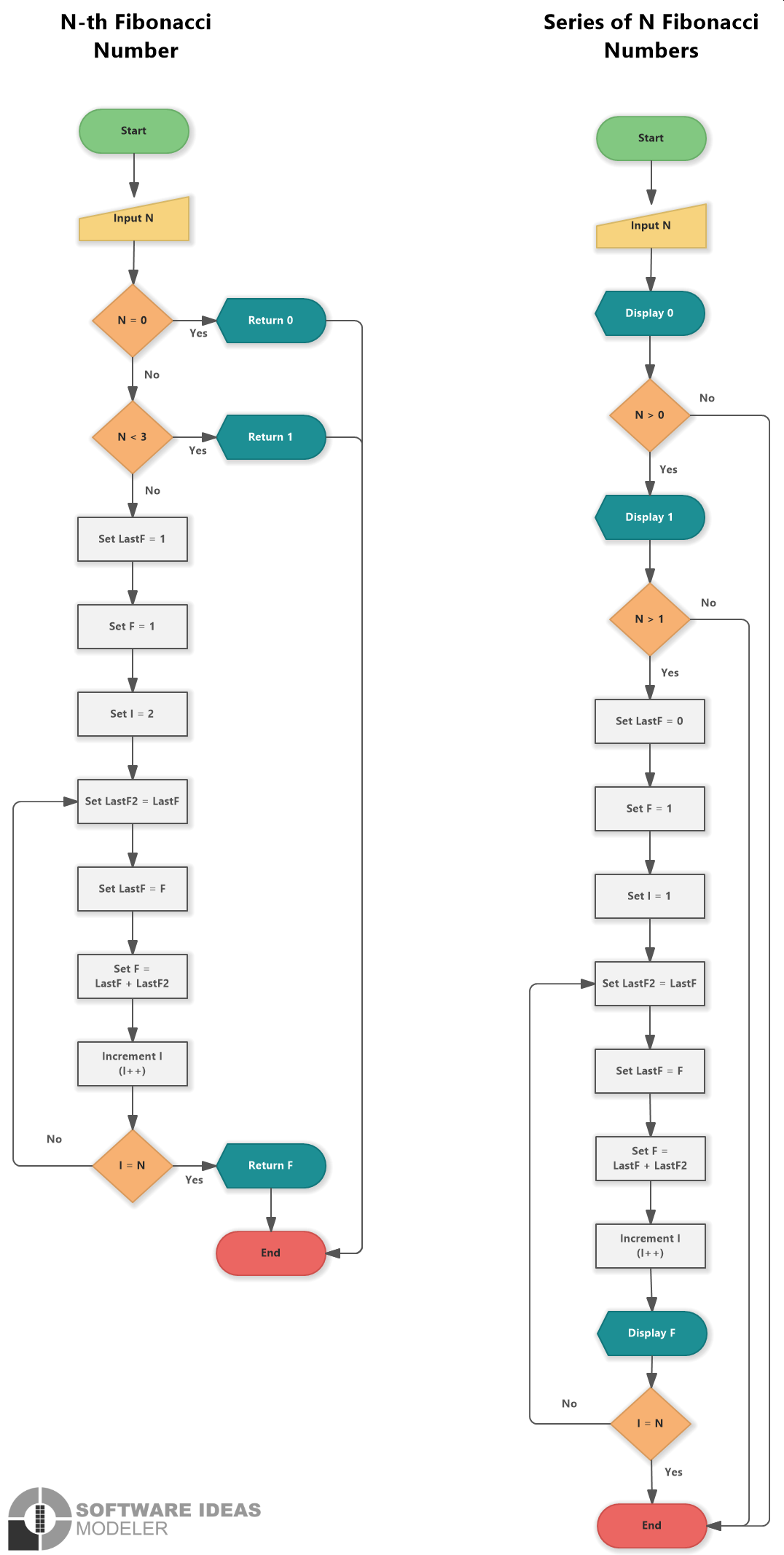# Fibonacci Series (Flowchart)

This flowchart example depicts an algorithm for calculating the Fibonacci number for N.

## Flowchart for N-th Fibonacci Number and Fibonacci Series

The Fibonacci number for 0 is defined as 0, for 1 as 1. Other subsequent numbers are defined as the sum of the two previous ones - 1 (0 + 1), 2 (1 + 1), 3 (1 + 2), 5 (2 + 3), 8 (3 + 5), ...

The first flowchart shows how to calculate N-th Fibonacci number. The algorithm shows the following steps:

• Start
• Input N (User input)
• N = 0 (Decision)
• Yes
• Return 0
• End
• N < 3 (Decision)
• Yes
• Return 1
• End
• Set LastF = 1
• Set F = 1
• Set I = 2
• Set LastF2 = LastF (Loop start)
• Set LastF = F
• Set F = Last F + LastF2
• Increment I (I++)
• I = N (Decision)
• Yes
• Return F
• End
• NoFlowchart for Fibonacci Series

The second flowchart depicts the steps for calculation of N Fibonacci numbers and displays them to the output. The algorithm consists of the following steps:

• Start
• Input N (User input)
• Display 0
• N > 0 (Decision)
• No
• End
• Display 1
• N > 1 (Decision)
• No
• End
• Set LasstF = 0
• Set F = 1
• Set I = 1
• Set LastF2 = LastF (Loop Start)
• Set LastF = F
• Set F = Last F + LastF2
• Increment I (I++)
• Display F
• I = N (Decision)
• No